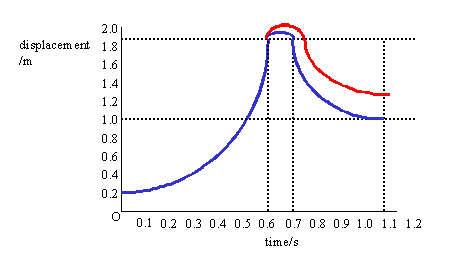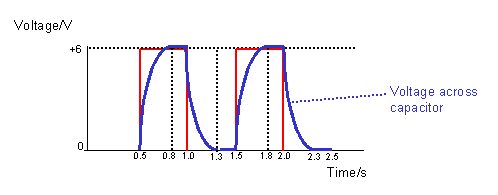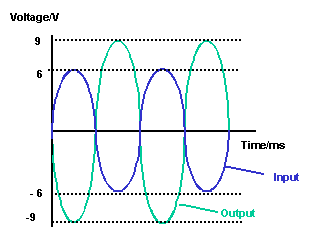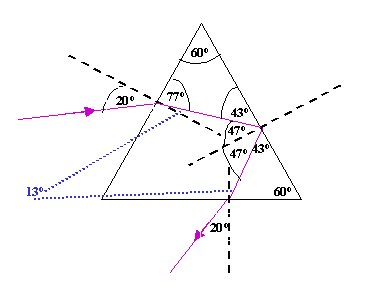# Solutions to SQA examination

## 2002

```Section A
1. C		11. B
2. A		12. D
3. A		13. B
4. C		14. E
5. E		15. E
6. C		16. A
7. A		17. E
8. C		18. D
9. B		19. D
10.B		20. E

Section B

21.a.i.  Initial displacement(s1) from sensor = 0.2m

a.ii.  Final displacement(s2) from sensor   = 1.8m

Ds =  s2 - s1
Ds =  1.8 - 0.2
Ds =  1.6m

a.iii. s = 1.6	s = ut + (at2)/2
u = 0m/s	s = (at2)/2
t = 0.6s	a = 2s/t2
a = ?		a = 2x1.6/0.62
a = 3.2/0.36
a = 8.9m/s2   (as required)

b.	Mean = total/N
Mean(a) = (8.9+9.1+8.4+8.5+9.0)/5
Mean(a) = (43.9)/5
Mean(a) = 8.78m/s2

Random error = (max-min)/N
Random error(a) = (9.1-8.4)/5
Random error(a) = (9.1-8.4)/5
Random error(a) = (0.7)/5
Random error(a) = 0.14m/s2

a = (8.8+-0.1)m/s2

NB: It is only useful to quote the final mean and
error to the same number of decimal places as
the least accurate individual measurement.

d.i. The contact time is greater with the softer surface.
ii.The rebound height will be less because the sponge
will absorb more of the balls kinetic energy.
The reduced rebound height can also be explained
as a result of the average upward force exerted by
the sponge on the ball being less.
iii. As the ball will sink more into the sponge the
maximum displacement from the sensor will be
greater.

22.a.	P1 = 109kPa
T1 = (15+273)K = 288K

P2 = ?
T2 = (45+273)K = 318K

P1/T1 = P2/T2
P2 = P1T2/T1
P2 = 109x318/288
P2 = 120.35kPa

b.   As the temperature increases the nitrogen gas molecules gain
kinetic energy. With increased kinetic energy the atoms are moving
faster and collide with the container walls more frequently
and forcefully. The pressure(force/area) therefore increases.

c.i.	P = 1.75x105Pa
A = 4.0x10-6m2

F = PA
F = 1.75x105x4.0x10-6
F = 0.7N

c.ii. Read from the graph the length of the spring when the force
is 0.7N.

Length = 35mm

d.	The assumption in the original set up is that the temperature
of the water is the same as the temperature of the gas inside
a more direct and accurate reading of the gas temperature.

23.a.	Use the law of conservation of momentum to solve this problem.
Pbefore = Pafter

Before collision
Pbefore = mvehicleuvehicle + mprobeuprobe
Pbefore = 2500x0.5 + 1500xuprobe
Pbefore = (1250+1500uprobe)kgm/s

After collision
Pafter = (mvehicle+mprobe)v
Pafter = (2500 + 1500)0.2
Pafter = 800kgm/s

(1250+1500uprobe) = 800	(by conservation of momentum)
1500u = 800-1250
1500u = -450
u = -450/1500

u = -0.3m/s		The negative indicates the probe
is initially moving in the opposite
direction to the vehicle.

b.i.	The direction of thrust from the engine must be opposite
to the direction of motion. This means it must be the
probe rocket engine that was switched on. (Favg = -500N)
NB: 	The average force has a negative value because
it is acting towards the left.

b.ii.	Favgt = DP
Favgt = (mv-mu)
Favgt = m(v-u)
t = m(v-u)/Favg
t = [4000(0-0.2)]/-500
t = 1.6s

c.	The initial acceleration to the RHS must be produced
by the space vehicle rocket engine. To then decelerate
the combined mass of the probe and vehicle the space
probe rocket engine must fire. In the maneuver the
space probe rocket must fire for twice the time of the
vehicle rocket because it only produces half the thrust.

Mathematically:
Fprobetprobe = Fvehicletvehicle
500tprobe=1000tvehicle
tprobe = 2tvehicle

24.a.	An emf of 6V means the battery will supply 6J of energy
to each coulomb of charge passing through it.

b.i.	emf = Vr + VR1 + VR2
emf = Ir + IR1 + IR2

I = 200mA = 0.2A

R2  = (emf - Ir - IR1)/I
R2  = (6.0 - 0.2x2.0 - 0.2x20)/0.2
R2  = (1.6)/0.2
R2  = (1.6)/0.2
R2  = 8W

b.ii. Vtpd = emf - Vr
Vtpd = emf - Ir
Vtpd = 6.0 - 0.2x2
Vtpd = 5.6V

c.	When the switch is closed a larger current is drawn from the
battery. This increases the voltage drop across the 2.0W
resistor. This voltage drop, called the "lost volts", therefore
increases. Consequently, the voltmeter reading, equal to:
emf - "lost volts", decreases.

25.a.i.	Initially all the supply voltage is across the resistor.
VR =  Vsupply = 6V

When the capacitor is fully charged: Vsupply = Vcapacitor
Vcapacitor = 6V

a.ii. E = CV2/2
E = (2000x10-6x62)/2
E = 0.072/2
E = 0.036J

a.iii.	Imax = Vsupply/R
R = Vsupply/Imax
R = 6/7.5x10-3
R = 800W

b.26.a.	Period(T) = 4cm x 2ms/cm
T = 8ms

f = 1/T
f = 1/8x10-3
f = 125Hz

b.i.	Inverting mode.

b.ii.The output voltage from the amplifier is calculated using:

Voutput/Vinput = -Rfeedback/Rinput
=>	Voutput = -Vinputx(Rfeedback/Rinput)
Voutput(peak) = -6x(3.0x103/2.0x103)
Voutput(peak) = -9V

(A)(B)	VRMS = Vpeak/SQRT2
VRMS = 9/1.414
VRMS = 6.36V

b.iii. As Rfeedback is increased the amplifier saturates. This means the
peak output voltage increases to a maximum of about +/- 13V.

27.a.	nglass = sinqair/sinqglass
nglass = sin20o/sin13o
nglass = 1.52

b.	The critical angle is the angle,measured between the ray
and the normal, at which light striking the glass air
boundary will be totally internally reflected.

c.	qcritical = sin-1(1/n)
qcritical = sin-1(1/1.52)
qcritical = sin-1(0.658)
qcritical = 41.1o

c.iii.28.a.	Threshold frequency.

28.b.i.	f0 = 3.33x1014Hz
work function(f) = hf0
f = 6.63x10-34x3.33x1014
f = 2.21x10-19J

b.ii.	 Ephoton = hf
Ephoton = 6.63x10-34x 5.66x1014
Ephoton = 3.75x10-19J

Ek(electron) = Ephoton - f
Ek(electron) = 3.75x10-19 - 2.21x10-19
Ek(electron) = 1.54x10-19J

b.iii. Ek(gain) = qDV
q = e = 1.6x10-19C
DV = 2.00x104V

Ek(gain) = 1.6x10-19 x 2.00x104
Ek(gain) = 3.2x10-15J

29.a.	Adding the impurity will decrease the resistance.

b.i.	When the electrons in the n-type material combine with
holes in the p-type material,as they cross the junction,
they lose energy. This energy is emitted as quanta of

ii.	dsinq = nl

d = 5.0x10-6m
q = 11o
n = 2
l = ?

l = dsinq/n
l = 5.0x10-6sin11o/2
l = 4.77x10-7m
l = 477nm

30.a.	Start by calculating the mass of pure torbernite(mtorbernite) in
the 0.6kg of material.

mtorbernite = (40/100)x0.6
mtorbernite = 0.24kg

The problem can now be solved by proportion.

MASS  :	ACTIVITY
1.0kg : 5.9x106decays per second = 5.9x106Bq = 5.9Mbq
0.24kg: 0.24x5.9Mbq

0.24kg has an activity of 1.416Mbq

b.	Alpha

H = QD
H = (20x150)mSv
H = 3000mSv

H/t = 3000/6
H/t = 500mSv/h.............1

Other
H   = (Qx400)mSv
H/t = (Qx400/8)mSv/h
H/t = (Qx50)mSv/h..........2

Equate 1 & 2

500mSv/h = (Qx50)mSv/h
Q = 500/50
Q = 10

This radiation must be fast neutrons.

END OF QUESTION PAPER